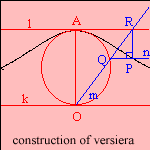# hyperbolism

## main

last updated: 2003-07-19

Given a curve C1, a point O, and a line l, then the hyperbolism of C1 is the curve C2 formed by the points P as follows:

• let Q be a point on C1, then line m through OQ intersects l in R
• let n be a line parallel to line l through Q
• then P is the projection from R on mLook at the witch of Agnesi to illustrate the construction:

We can normalize the hyperbolism to a curve C1 with respect to a point O and a line x = 1.
In this case holds that when C1 has equation f (x,y) = 0, the hyperbolism of C1 has as equation: f (x, xy) = 0.
The term xy makes the link to the hyperbola.

The inverse of the hyperbolism is the antihyperbolism: when C2 is the hyperbolism of C1, then C1 is the antihyperbolism of C2.

Some examples are the following:
 C1 O wrt C1 l wrt C1 l wrt C2 O wrt C2 C2 circle on circle parallel to diameter through O - center serpentine circle on circle tangent on opposite side of O parallel to asymptote center witch of Agnesi circle center tangent Kulp's quartic